## How to Calculate and Solve for Exposure Rate of Chemicals | Material Selection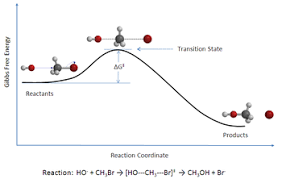The image above represents exposure rate of chemical.

To compute for exposure rate of chemical, four essential parameters are needed and these parameters are Diffusion Coefficient (D), Area of Contact (A), Safety Factor (SA) and length (l).

The formula for calculating exposure rate of chemical:

re = DASA/l

Where:

re = Exposure Rate of Chemicals
D = Diffusion Coefficient
A = Area of Contact
SA = Safety Factor
l = Length

Let’s solve an example;
Find the exposure rate of chemicals when the diffusion coefficient is 10, the area of contact is 14, the safety factor is 12 and the length is 8.

This implies that;

D = Diffusion Coefficient = 10
A = Area of Contact = 14
SA = Safety Factor = 12
l = Length = 8

re = DASA/l
re = (10)(14)(12)/8
re = 1680/8
re = 210

Therefore, the exposure rate of chemicals is 210.

Calculating the Diffusion Coefficient when the Exposure Rate of Chemicals, the Area of Contact, the Safety Factor and the Length is Given.

D = re x l / ASA

Where;

D = Diffusion Coefficient
re = Exposure Rate of Chemicals
A = Area of Contact
SA = Safety Factor
l = Length

Let’s solve an example;
Find the diffusion coefficient when the exposure rate of chemicals is 20, the area of contact is 8, the safety factor is 4 and the length is 2.

This implies that;

re = Exposure Rate of Chemicals = 20
A = Area of Contact = 8
SA = Safety Factor = 4
l = Length = 2

D = re x l / ASA
D = 20 x 2 / 8(4)
D = 40 / 32
D = 1.25

Therefore, the diffusion coefficient is 1.25.

## How to Calculate and Solve for Chemical Breakthrough Time | Material Selection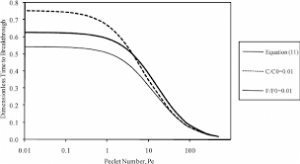The image above represents chemical breakthrough time.

To compute for chemical breakthrough time, two essential parameters are needed and these parameters are Thickness (l) and Diffusion Coefficient (D).

The formula for calculating chemical breakthrough time:

tb = /6D

Where:

tb = Chemical Breakthrough Time
l = Thickness
D = Diffusion Coefficient

Let’s solve an example;
Find the chemical breakthrough time when the thickness is 14 and the diffusion coefficient is 7.

This implies that;

l = Thickness = 14
D = Diffusion Coefficient = 7

tb = / 6D
tb = 14²/6(7)
tb = 196/42
tb = 4.66

Therefore, the chemical breakthrough time is 4.66 s.

Calculating the Thickness when the Chemical Breakthrough Time and the Diffusion Coefficient is Given.

l = √tb x 6D

Where;

l = Thickness
tb = Chemical Breakthrough Time
D = Diffusion Coefficient

Let’s solve an example;
Find the thickness when the chemical breakthrough time is 5 and the diffusion coefficient is 20.

This implies that;

tb = Chemical Breakthrough Time = 5
D = Diffusion Coefficient = 20

l = √tb x 6D
l = √5 x 6(20)
l = √5 x 120
l = √600
l = 24.49

Therefore, the thickness is 24.49.

## How to Calculate and Solve for Tensile Strength of Alloy of Spring | Material Selection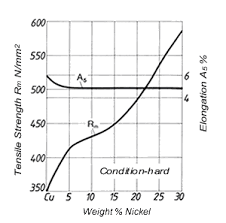The image above represents tensile strength of alloy of spring.

To compute for tensile strength of alloy of spring, one essential parameter is needed and this parameter is Cross-section Diameter of Spring (d).

The formula for calculating tensile strength of alloy of spring:

TS(psi) = 169000(d)-0.167

Where:

TS(psi) = Tensile Strength of Alloy of Spring
d = Cross-section Diameter of Spring

Let’s solve an example;
Find the tensile strength of alloy of spring whose cross-section diameter of spring is 24.

This implies that;

d = Cross-section Diameter of Spring = 24

TS(psi) = 169000(d)-0.167
TS(psi) = 169000(24)-0.167
TS(psi) = 169000(0.58)
TS(psi) = 99401.15

Therefore, the tensile strength of alloy of spring is 99401.15.

## How to Calculate and Solve for Goodman’s Law | Material Selection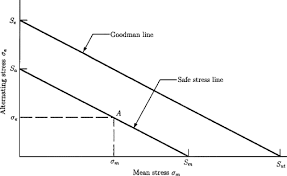The image above represents Goodman’s law.

To compute for goodman’s law, three essential parameters are needed and these parameters are Fatique Limit (τe), New Fatique Limit (τm) and Tensile Strength of Alloy of Spring (TS).

The formula for calculating Goodman’s law:

τ = τe(1 – τm/0.67TS)

Where:

τ = Goodman’s Law (τ)
τe = Fatique Limit
τm = New Fatique Limit
TS = Tensile Strength of Alloy of Spring

Let’s solve an example;
Find the goodman’s law when the fatique limit is 4, the new fatique limit is 10 and the tensile strength of alloy of spring is 12.

This implies that;

τe = Fatique Limit = 4
τm = New Fatique Limit = 10
TS = Tensile Strength of Alloy of Spring = 12

τ = τe(1 – τm/0.67TS)
τ = 4(1 – 10/0.67(12))
τ = 4(1 – 10/8.040)
τ = 4(1 – 1.24)
τ = 4(-0.24)
τ = -0.975

Therefore, the goodman’s law is -0.975.

## How to Calculate and Solve for Deflection of Coil of Spring | Material Selection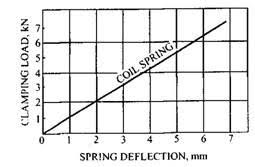The image above represents deflection of coil of spring.

To compute for deflection of coil of spring, two essential parameters are needed and these parameters are Total Spring Deflection (σs) and Number of Effective Spring Coils (Nc).

The formula for calculating deflection of coil of spring:

σc = σs/Nc

Where:

σc = Deflection of Coil of Spring (Nc)
σs = Total Spring Deflection
Nc = Number of Effective Spring Coils

Let’s solve an example;
Find the deflection of coil of spring when the total spring deflection is 24 and the number of effective spring coils is 12.

This implies that;

σs = Total Spring Deflection = 24
Nc = Number of Effective Spring Coils = 12

σc = σs / Nc
σc = 24/12
σc = 2

Therefore, the deflection of coil of spring is 2 m.

Calculating the Total Spring Deflection when the Deflection of Coil of Spring and the Number of Effective Spring Coils is Given.

σs = σc x Nc

Where;

σs = Total Spring Deflection
σc = Deflection of Coil of Spring (Nc)
Nc = Number of Effective Spring Coils

Let’s solve an example;
Find the total spring deflection when the deflection of coil of spring is 10 and the number of effective spring coils is 5.

This implies that;

σc = Deflection of Coil of Spring (Nc) = 10
Nc = Number of Effective Spring Coils = 5

σs = σc x Nc
σs = 10 x 5
σs = 50

Therefore, the total spring deflection is 50.

## How to Calculate and Solve for Deflection of Coil of Spring | Material Selection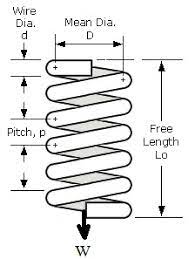The image above represents deflection of coil of spring.

To compute for deflection of coil of spring, four essential parameters are needed and these parameters are Force (F), Centre to Centre Diameter of Spring (D), Cross-section Diameter of Spring (d) and Shear Modulus (G).

The formula for calculating deflection of coil of spring:

σc = 8FD³/d4G

Where:

σc = Deflection of Coil of Spring (G)
F = Force
D = Centre to Centre Diameter of Spring
d = Cross-section Diameter of Spring
G = Shear Modulus

Let’s solve an example;
Find the deflection of coil of spring when the force is 8, the centre to centre diameter of spring is 2, the cross-section diameter of spring is 4 and the shear modulus is 6.

This implies that;

F = Force = 8
D = Centre to Centre Diameter of Spring = 2
d = Cross-section Diameter of Spring = 4
G = Shear Modulus = 6

σc = 8FD³/d4G
σc = 8(8)(2)³/(4)4(6)
σc = 8(8)(8)/(256)(6)
σc = 512/1536
σc = 0.33

Therefore, the deflection of coil of spring is 0.33 m.

## How to Calculate and Solve for Force Independent Constant | Material Selection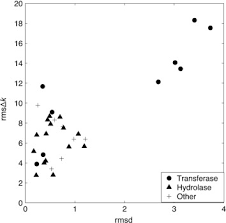The image above represents force independent constant.

To compute for force independent constant, two essential parameters are needed and these parameters are Centre to Centre Diameter of Spring (D) and Cross-section Diameter of Spring (d).

The formula for calculating force independent constant:

Kw = 1.60(D/d)-0.14

Where:

Kw = Force Independent Constant
D = Centre to Centre Diameter of Spring
d = Cross-section Diameter of Spring

Let’s solve an example;
Find the force independent constant when the centre to centre diameter of spring is 44 and the cross-section diameter of spring is 11.

This implies that;

D = Centre to Centre Diameter of Spring = 44
d = Cross-section Diameter of Spring = 11

Kw = 1.60(D/d)-0.14
Kw = 1.60(44/11)-0.14
Kw = 1.60(4)-0.14
Kw = 1.60(0.82)
Kw = 1.317

Therefore, the force independent constant is 1.317.

## How to Calculate and Solve for Spring Torque | Material Selection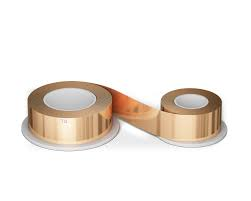The image above represents spring torque.

To compute for spring torque, three essential parameters are needed and these parameters are Force Independent Constant (Kw), Compressive Force (F), Centre to Centre Diameter of Spring (D) and Cross-section Diameter of Spring (d).

The formula for calculating spring torque:

τ = Kw(8FD/πd³)

Where:

τ = Spring Torque
Kw = Force Independent Constant
F = Compressive Force
D = Centre to Centre Diameter of Spring
d = Cross-section Diameter of Spring

Let’s solve an example;
Find the spring torque when the force independent constant is 12, the compressive force is 24, the centre to centre diameter of spring is 11 and the cross-section diameter of spring is 44.

This implies that;

Kw = Force Independent Constant = 12
F = Compressive Force = 24
D = Centre to Centre Diameter of Spring = 11
d = Cross-section Diameter of Spring = 44

τ = Kw(8FD/πd³)
τ = 12(8(24)(11)/π(44)³)
τ = 12(2112/π(85184))
τ = 12(2112/267613.428)
τ = 12(0.0078)
τ = 0.0947

Therefore, the spring torque is 0.0947 Nm.

## How to Calculate and Solve for Stiffness Performance Index | Material Selection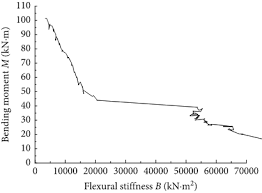The image above represents stiffness performance index.

To compute for stiffness performance index, two essential parameters are needed and these parameters are Shear Modulus (G) and Density (ρ).

The formula for calculating stiffness performance index:

P = √(G)/ρ

Where:

P = Stiffness Performance Index
G = Shear Modulus
ρ = Density

Let’s solve an example;
Find the stiffness performance index when the shear modulus is 36 and the density is 12.

This implies that;

G = Shear Modulus = 36
ρ = Density = 12

P = √(G)/ρ
P = √(36)/12
P = 6/12
P = 0.5

Therefore, the stiffness performance index is 0.5

Calculating the Shear Modulus when the Stiffness Performance Index and the Density is Given.

G = (P x ρ)2

Where:

G = Shear Modulus
P = Stiffness Performance Index
G = Shear Modulus= Stiffness Performance Index
ρ = Density

Let’s solve an example;
Find the shear modulus when the stiffness performance index is 25 and the density is 5.

This implies that;

P = Stiffness Performance Index = 25
ρ = Density = 5

G = (P x ρ)2
G = (25 x 5)2
G = 15,625

Therefore, the shear modulus is 15,625.

## How to Calculate and Solve for Performance Index | Material Selection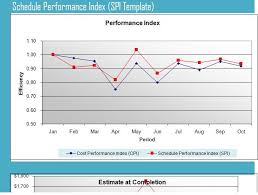The image above represents performance index.

To compute for performance index, two essential parameters are needed and these parameters are Shear Stress at Fracture (τf) and Density (ρ).

The formula for calculating the performance index:

P = τf2/3 / ρ

Where:

P = Performance Index
τf = Shear Stress at Fracture
ρ = Density

Let’s solve an example;/
Find the performance index when the shear stress at fracture is 32 and the density is 10.

This implies that;

τf = Shear Stress at Fracture = 32
ρ = Density = 10

P = τf2/3 / ρ
P = 322/3/10
P = 10.079 / 10
P = 1.0079

Therefore, the performance index is 1.0079.